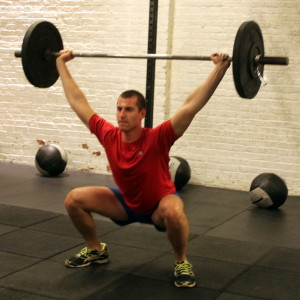## Friday, December 2, 2016

### Friday, December 2, 2016as many rounds as possible in 18 minutes:
200m run
21x squat
15x standing long jump, 6-ft.
9x ring dipas many rounds as possible in 18 minutes:
200m run
21x squat
15x standing long jump, 6-ft.
9x close-grip pushup

Post score to comments.

### 1 Comment

1.–Scores–
Al C.: 3 rounds + 100m run
Liz L.: 3 rounds + 5x slj (sub: 4′, 1/2x static dip negative)
Brad G.: 5 rounds + 9x slj
Borja G.: 5 rounds + 10x squat
John L: 3 rounds + 150m run (sub: 1/2x ring dip negative)
Walt C.: 4 rounds + 100m run (sub: 5′, 1/2x ring dip)
Anna G.: 4 rounds + 100m run(sub: 5′, 1/2x static dip negative)
Ralph A.: 3 rounds (sub: 5′, 1/2x ring dip)
Hari P.: 4 rounds + 9x squat
Andrew Z.: 4 rounds + 100m run
Kevin K.: 3 rounds + 7x slj (sub: 1/2x ring dip)
Kate H.: 3 rounds (sub: 5′, 1/2x static dip negative)
Christine S.: 4 rounds (sub: 5′)
Michael F.: 5 rounds
Greg K.: 4 rounds (sub: 1/2x ring dip)
David C.: 4 rounds + 21x squat (sub: 1/2x static dip, 2/3x slj)
Kaylan M.: 3 rounds (sub: 2/3x slj, 1/2x bench dip)
Chris C.: 5 rounds + 150m run (sub: deficit pushup)
Chase H.: 4 rounds + 9x slj (sub: 2/3x slj, 5′, 1/2x static dip)
Travers O.: 4 rounds + 9x slj (sub: 2/3x slj, 4′, 1/2x static dip)
Jess W.: 2 rounds + 3x slj (sub: 2/3x slj, 3.5′, 1/2x bench)
Sioban : 3 rounds + 8x slj (sub: 2/3x slj, 4′, 1/2x bench dip)
Josh W.: 2 rounds + 15x squat (sub: 2/3x slj, 4′, 1/2x bench dip)
Blaine P.: 4 rounds
David S.: 5 rounds
Alissa G.: 4 rounds (sub: 2/3x slj, pushup)
Mali P.: 4 rounds + 6x slj (sub: slj, 5′, 1/2x static dip negative)
Aaron R.: 4 rounds + 2x slj (sub: 1/2x ring dip)
Kevin M.: 3 rounds + 21x slj sub: 4′, 1/2x ring dip)
Joe L.: 4 rounds + 12x slj (sub: 1/2x ring dip)
Dave R.; 4 rounds + 12x squat
Diana D.: 3 rounds + 5x slj (sub: 5′, 1/2x bench hip)
Buffy M.: 4 rounds + 4x slj (sub: 5′, 1/2x static dip negative)
Will O.: 4 rounds + 100m run (sub: 1/2x ring dip)
Patrick J.: 4 rounds + 100m run (sub: 1/2x ring dip)
Jeff C.: 4 rounds + 100m run (sub: 5′, 1/2x ring dip)
Holmes H.: 4 rounds + 100m run (sub: 5′, 1/2x bench dip)
Cristian D.: 4 rounds (sub: 4′, 1/2x ring dip)
Ben A.: 4 rounds + 5 slj (sub: 5′)
James H.: 5 rounds (sub: 15x max vertical jump)
Josh M.: 4 rounds + 8x slj (sub: 5′, 5/3x pushup)
Kat S.: 4 rounds (sub: 5′, static dip negatives)
Diana G.: 4 rounds (sub: 2/3x sls, 4′, true bench dip)
Elise C.: 4 rounds + 45” airdyne (sub: 1′ airdyne, 5′, 1/2x static dip)
Josh B.: 4 rounds + 12x slj (sub: 15x max vertical jump)
Tara S.: 4 rounds (sub: 5′)
Francisco L.: 4 rounds + 3 ring dip (sub: 5′, 1/2x ring dip)
Saumil S.: 4 rounds
Will H.: 4 rounds (sub: 1/2x ring dip)
Mark Co.: 4 rounds + 100m run
Sean Mc.: 4 rounds + 3x squat
Amelia F.: 4 rounds (sub: 5′, static dip negative)
Peter M: 4 rounds + 13x slj (sub: 1/2x ring dip)